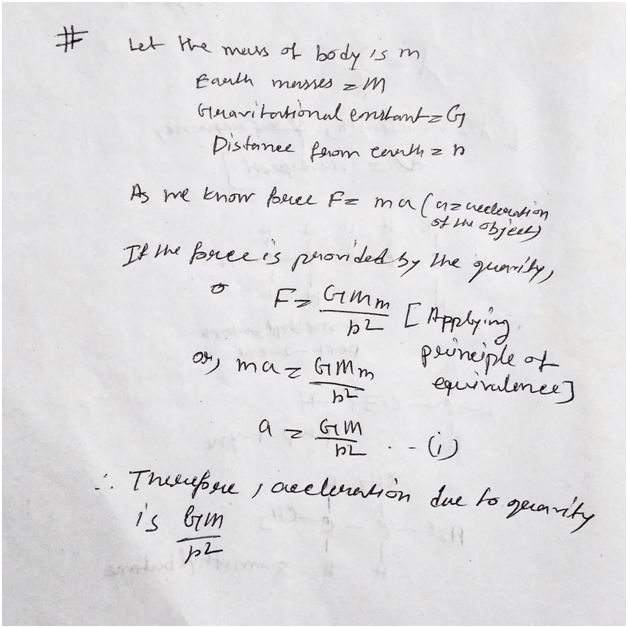# Chhattisgarh State Class 9 Science Chapter 11 Solution

Chhattisgarh State Board Class 9 Science Chapter 11 Gravitation Exercise Multiple Choice, Fill in the Blanks, Questions and Answers here.

## Chhattisgarh State Class 9 Science Chapter 11 Solution

1) Multiple choice questions-

(i) The gravitational force between two bodies does not depend on-

(a) The distance between both the bodies.

(b) Product of their masses

(c) Sum of their masses

(d) Gravitational constant.

Ans: – option (c).

(ii) The value of G is-

(a) 7.67 × 1011Nm2/kg2

(b) 6.67 × 1011Nm2/kg

(c) 6.67 × 10–11Nm2/kg2

(d) 5.67 × 1011Nm2/kg2.

Ans: – option (c).

(iii) The value of gravitational acceleration on the surface of earth is-

(a) 9.8 m/s2

(b) 8.8 m/s2

(c) 4.8 m/s2

(d) 8.9 m/s2.

Ans: – option (a).

(iv) According to universal law of gravitation, the force acting between two bodies of massesm1 and m2 placed at a distance R is-

(a) F=Gm1m2/R^2.

(b) F=Gm1m2/R^4.

(c) F = Gm1m2/R.

(d) F=GM/R.

Ans: – option (a).

(v) A body is moving upwards opposing the gravitational pull of earth. What will be its velocityat the highest point reached?

(a) 0 (b) u2/2g

(c) h/t (d) 2gh.

Ans: – option (a).

2) Fill in the blanks-

(i) On earth, the weight of a body having 10 kg. mass will be ……….1kg………….

(ii) A body falling from rest from a height h will have a velocity of ……….0…………

(iii) Value of universal gravitational constant is ……6.67×10^-11 Nm^2/kg^2…………………..

(iv) The value of gravitational acceleration in SI units is ………9.8m/s^2……….

(v) If two objects of different masses are dropped from a same height, they will reach thesurface of earth at……same…………………….. time.

3) What will be the gravitational force between earth and an object of 1 kg. placed on its surface.Here, the mass of earth is 6×1024 kg, and distance from the earth to the earth’s surface is 6400 km.

Ans: – As we all know that, F=GMm/r^2.

Here G= gravitational constant=6.67×10^-11; M=earth mass= 6×10^24kg; m=mass of the object= 1kg; R=6400.

So, F= 6.67×10^-11 × 6×10^24 × 1 /(6400)^2 = 9.77N.

4) What will be the gravitational force between two objects if-

(i) The mass of one object is doubled.

(ii) The distance between the objects is tripled.

(iii) Mass of both the objects is doubled.

Ans: – We know that gravitational force F=Gm1m2/R^2;

For case (i), If the mass of one object is double then m1 or m2 will be double so the value of forces will be double.

For case (ii), if the distance between them R is triple then the gravitational forces between them will be 1/9 times of its previous value.

Ans for case (iii) if the mass of both object is doable then the gravitational force between them will be four times as comparison to previous value.

5) Why does a sheet of paper fall slower than a ball made up of that sheet.

Ans: – If the both paper and the ball made off with the paper dropped from same height then we will see that the paper falls slowly. This phenomenon happened because of the large surface are of the paper as compare to the ball so the air resistance is varying.

6) What is the importance of the universal law of gravitation.

Ans: – The importance of the gravitation is huge. For this law the substance in the earth are stayed in its position. This law also helps the launching of the satellite from the earth surface. The escape velocity must be covered while launching the satellite from the earth.

7) Why does earth not travel towards the moon, if moon attracts the earth?

Ans: – The force of attraction between the moon and earth causes the moon travel outside the earth. As the force of attraction between the earth to moon is more than the force of attraction between the moon to earth moon moving around the earth.

8) A ball is thrown vertically upwards with a speed of 49 m/s. Calculate-

(i) Maximum height of the ball

(ii) Total time taken by the ball to reach the earth’s surface.

Ans: – As we all know that, V^2=u^2 + 2aS;

Here, V=0; u=49 m/s; a=g= 10m/s^2;

So, S=120.5m.

Then the height of the ball is 120.5m.

And, V= u+ at; or, t=-(u/a);

So, t= 49/10= 4.9s.

Then the total time will be 4.9×2=9sec.

9) If a body is thrown vertically upwards at a speed of 10 m/s then after how much time and with whatvelocity will it reach back to us?

Ans: – As we all know, v^2 = u^2 + 2as; Here v=0; u=10m/s.

A=g=10m/s^2;

So, s=-(100/20) =-50m (negative sign represent opposite direction).

And, v= u + at; or, t=50/10 = 5sec.

So, the total time will be 2×5=10sec.

For downward direction,

U=0; v=? Ant t=5sec.

So, v= u + at; or, v= 50m/s.

10) The force of gravitation between two bodies is F. In what situation will the force between the two become 4 F?

Ans: – As we all know, F=Gm1m2/R^2;

So, this F will be 4F in case if the masse of this object is double, or the distance is half etc.

11) Why do two objects having different mass reach the surface of earth simultaneously? Is the force of gravitation acting on both of them equal?

Ans: – The two different objects of different masses if dropped from any height then we will see that both object falls to the ground in same time. As the force of gravitation acting between this two are same the object touches the ground at same times.

12) Derive an equation to find the acceleration produced due to earth on an object of mass ‘m’. Also find its value.

Ans: –Updated: January 6, 2022 — 3:12 pm